# Quiz 3: Opportunity Cost of Capital and Capital Budgeting

Business

Capital Budgeting Capital budgeting is the process used by the company to evaluate the capital investment that are available. The company uses various capital budgeting methods to evaluate whether the company should invest in the particular project that is whether the project is profitable for the company. Internal rate of return This is the rate of return at which the net present value of the project is zero that is any return above this would give positive NPV and any return below this would give negative NPV. Use the below formula to calculate internal rate of return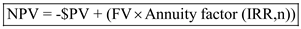Net present value is NPV which is zero at IRR Present value is PV Future value is FV Internal rate of return is IRR Present value Present value calculates the value of money today which is to be received in future date. It calculates how much the future value is worth today. Present value can be calculated using the present value table or present value formula.Formula to calculate present value of lump sum amount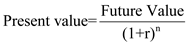Interest rate is r Time period is n Calculate internal rate of return on this investment as shown belowUsing the present value of annuity table we get IRR at 4%, in this table under 7 look for annuity factor of 6.002 which would be given for i=4% Thus, IRR for this investment is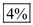Capital Budgeting Capital budgeting is the process used by the company to evaluate the capital investment that are available. The company uses various capital budgeting methods to evaluate whether the company should invest in the particular project that is whether the project is profitable for the company. Depreciation Depreciation is writing down the value of assets to income statement over the useful life of assets. The assets purchased are initially capitalized to the balance sheet as the entire cost of asset would be used over the years and so debiting the entire amount of asset purchase would lead to wrong reporting of net income for current and subsequent years. Since the asset would be used over the years, the cost will also be recognized over the years in the income statement. This cost is known as depreciation. Depreciation is a non cash expense which would not impact the cash of the company. There are different methods to calculate depreciation which are straight line method, declining balance method, sum of digits method and so on. In case the taxable income is positive and tax rate is constant for each year then accelerated method of depreciation is preferable rather than straight line. In case of accelerated method, depreciation is calculated on declining balance method and thus depreciation charged for earlier years would be higher than for later years. The original value of asset would be written off earlier in accelerated method rather than straight line method. This would provide for higher income tax shield under accelerated method rather than straight line method in earlier time period of project. The present value of tax shield under accelerated method would therefore be higher than under straight line method. In such case accelerated method would be preferred over straight line method.

Capital Budgeting Capital budgeting is the process used by the company to evaluate the capital investment that are available. The company uses various capital budgeting methods to evaluate whether the company should invest in the particular project that is whether the project is profitable for the company. Internal rate of return This is the rate of return at which the net present value of the project is zero that is any return above this would give positive NPV and any return below this would give negative NPV. Use the below formula to calculate internal rate of returnNet present value is NPV which is zero at IRR Present value is PV Future value is FV Internal rate of return is IRR Net present value Net present value refers to present value of cash inflow less present value of cash outflow. This considers the cash inflow and outflow and also considers time value of money. Use the below formula to calculate net present value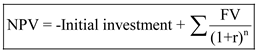Discount rate is r Time period is n Present value Present value calculates the value of money today which is to be received in future date. It calculates how much the future value is worth today. Present value can be calculated using the present value table or present value formula.Formula to calculate present value of lump sum amountInterest rate is r Time period is n a.Calculate internal rate of return for investment A as shown belowUsing the present value of annuity table we get IRR at 20%, in this table under 5 look for annuity factor of 2.991 which would provide i=20% Thus, IRR for investment A is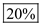Calculate internal rate of return for investment B as shown belowUsing the present value of annuity table we get IRR at 25%, in this table under 5 look for annuity factor of 2.689 which would provide i=25% Thus, IRR for investment B is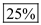b. Both the investment have IRR above 15% and based on IRR both should be accepted. In order to choose one investment we would calculate NPV of both project and investment with higher NPV would be selected.Calculate net present value of investment A as shown belowThus, NPV of investment A is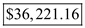Calculate net present value of investment B as shown belowThus, NPV of investment B is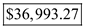The NPV of investment B is higher than investment A and thus investment B is preferable.